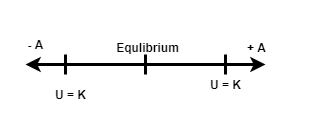# Problem: A harmonic oscillator has angular frequency ω and amplitude A.(a) How often is elastic potential energy is equal to the kinetic energy in each cycle?(b) What is the time between occurrences?

###### FREE Expert Solution

Angular frequency:

$\overline{){\mathbf{\omega }}{\mathbf{=}}{\mathbf{2}}{\mathbf{\pi }}{\mathbf{f}}}$In the above diagram, when moving from -A to +A and back to -A, we'll encounter 4 points where the elastic potential energy is equal to the kinetic energy

82% (22 ratings)###### Problem Details

A harmonic oscillator has angular frequency ω and amplitude A.

(a) How often is elastic potential energy is equal to the kinetic energy in each cycle?

(b) What is the time between occurrences?